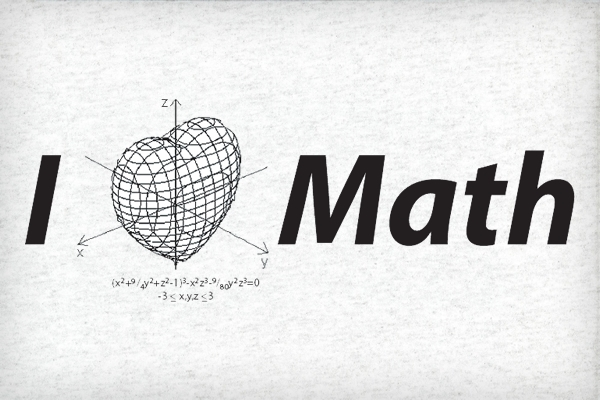The Collatz Conjecture is one of the Unsolved problems in mathematics, especially in Number Theory. The Collatz Conjecture is also termed as 3n+1 conjecture, Ulam Conjecture, Kakutani’s Problem, Thwaites Conjecture, Hasse’s Algorithm, Syracuse Problem.

### Statement:

Halve it, if it is even.
Or
triple it and add 1, if it is odd.

If you keep repeating this procedure, you shall reach the number 1 at last.

### Illustrations

» Starting with 1 — we get 1 in first step.
» Starting with 2 (even) — we get 1 in second step and in one operation $2 \to 1$
» Starting with 3 (odd) — we get 1 in 8th step $3 \to 10 \to 5 \to 16 \to 8 \to 4 \to 2 \to 1$

Similarly, you can check this conjecture for every positive integer; you should get 1 at last according to this conjecture.

### Mathematical Illustration

Let $\mathbf {n}$ be a positive integer. Then it either be even or odd.
A. If n is even: Divide $\mathbf {n}$ by $\mathbf {2}$ and get $\mathbf {\frac {n}{2} }$ . Is it 1? — conjecture applies on that positive integer. Again if it is even — redo the same work. If it is odd, then— see next step!
B. If n is odd: Multiply $\mathbf n$ by $\mathbf 3$ & then add $\mathbf 1$ to find $\mathbf 3n+1$ . Is it 1? — conjecture applies on that positive integer. Again if it is even — redo the same work you did in A. If it is odd, then— redo the work of B!

### Problem in this Conjecture

This conjecture has been tried on various kind of numbers, and those numbers have satisfied the Collatz Conjecture. But the question is that —

Is this conjecture applicable to every positive integer?

### Note

Mathematicians have found no good use of Collatz Conjecture in Mathematics, so it is considered as a useless conjecture. But overall, it is unsolved — and we can’t leave any unknown or unsolved problems & principles in Math.

Feel free to ask questions, send feedback and even point out mistakes. Great conversations start with just a single word. How to write better comments?
1.to.ly-ADU5 says:

Hey there! Quick question that’s totally off topic.

Do you know how to make your site mobile friendly?
My web site looks weird when viewing from my iPhone.
I’m trying to find a theme or plugin that might be able to correct this issue.
If you have any recommendations, please share.

Appreciate it!

2.shubham choudhary says:

I can solve collatz problem

## Hopalong Orbits Visualizer: Stunning WebGL Experiment

Just discovered Barry Martin’s Hopalong Orbits Visualizer — an excellent abstract visualization, which is rendered in 3D using Hopalong Attractor algorithm, WebGL and Mrdoob’s three.js project. Hop to the source website using your desktop browser (with WebGl and Javascript support) and enjoy the magic. PS: Hopalong Attractor Algorithm Hopalong Attractor predicts the locus of points in 2D using this algorithm…

## Understanding Poincaré Conjecture

Introduction & Statement of Poincaré Conjecture In 1904, the french Mathematician Henri Poincaré posed an epoch-making question in one of his papers, which asked: If a three-dimensional shape is simply connected, is it homeomorphic to the three-dimensional sphere? Explanation The statement can be explained by considering the analogous two-dimensional situation. Let us think of a rubber band stretched around the…

## How many apples did each automattician eat?

Four friends Matt, James, Ian and Barry, who all knew each other from being members of the Automattic, called Automatticians, sat around a table that had a dish with 11 apples in it. The chat was intense, and they ended up eating all the apples. Everybody had at least one apple, and everyone know that fact, and each automattician knew…

## Two Interesting Math Problems

Problem1: Smallest Autobiographical Number: A number with ten digits or less is called autobiographical if its first digit (from the left) indicates the number of zeros it contains,the second digit the number of ones, third digit number of twos and so on. For example: 42101000 is autobiographical. Find, with explanation, the smallest autobiographical number. Solution of Problem 1 Problem 2:…

## 381654729 : An Interesting Number Happened To Me Today

You might be thinking why am I writing about an individual number? Actually, in previous year annual exams, my registration number was 381654729. Which is just an ‘ordinary’ 9-digit long number. I never cared about it- and forgot it after exam results were announced. But today morning, when I opened “Mathematics Today” magazine’s October 2010, page 8; I was brilliantly…

## The Area of a Disk

If you are aware of elementary facts of geometry, then you might know that the area of a disk with radius $R$ is $\pi R^2$ . The radius is actually the measure(length) of a line joining the center of disk and any point on the circumference of the disk or any other circular lamina. Radius for a disk…